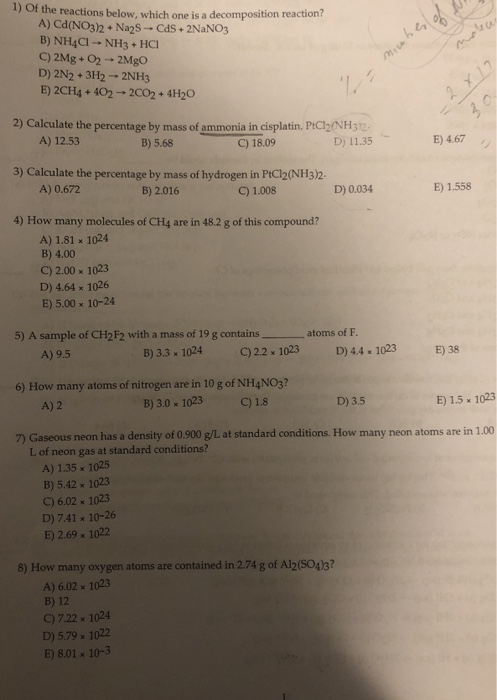# If a 1.5 V AAA alkaline battery can power a 1.4 Watt device for 1 hour,...

If a 1.5 V AAA alkaline battery can power a 1.4 Watt device for 1 hour, how many grams of zinc (65.38g/mol) does it contain?

Overall reaction in battery: MnO2 + 2H2O + Zn --> Mn(OH)2+ ZnO

The equation

P = I*V

then

I = P/V

P = 1.4 watt; V = 1.5

then

I = 1.4/1.5 = 0.9333 Amp

1 amp = 1 C/s = 0.9333 C/s

t = 3600 s so

0.9333*3600 = 3359.88 C

convert to mol of electrons

1 mol of e- = 96500 C

then

3359.88/96500 = 0.0348174 mol of electrons being transferred

MnO2 + 2H2O + Zn --> Mn(OH)2+ ZnO

Zn goes from 0 to +2 therefore

2 mol of e are being transferred

for every mol of Zn

0.0348174/2 --> 0.0174087mol of Zn

mass of Zn = mol*MW = 0.0174087*65.38 = 1.138180806 g of Zn

#### Earn Coin

Coins can be redeemed for fabulous gifts.

Similar Homework Help Questions
• ### During the discharge (operation) of a 1.5 alkaline battery, Robin determined that 6.33g of zinc was...

During the discharge (operation) of a 1.5 alkaline battery, Robin determined that 6.33g of zinc was oxidized to zn(oh)2. a) what mass of Mno2 was reduced at the cathode. b) How many coloumbs of electron charge we're transferred from zn to Mno2 ? c) How many joules of electrical energy we're produced ?

• ### if you can't answer all ,please don't answer 1) of the reactions below, which one is...if you can't answer all ,please don't answer 1) of the reactions below, which one is a decomposition reaction? A) Ca(NO3)2 + Na2S -- CdS +2NaNO3 B) NH4Cl- NH3 + HCI C) 2Mg + 02 - 2MgO D) 2N2 + 3H2 → 2NH3 E) 2CH4 + 402 - 2002 + 4H20 miches of - x 1? 2 2) Calculate the percentage by mass of ammonia in cisplatin, PtCl2/NH3 12. A) 12.53 B) 5.68 C) 18.09 D) 11.35 E) 4.67 3)...

Free Homework App